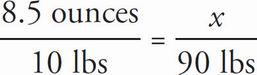# SAT Math Multiple Choice Question 85: Answer and Explanation

### Test Information

Question: 85

10. Dogs need 8.5 to 17 ounces of water each day for every 10 pounds of their weight. Everett has two dogs-Ringo is a 35-pound black lab mix, and Elvis is a 55-pound beagle. Which of the following ranges represents the approximate total number of ounces of water, w, that Elvis and Ringo need in a week?

• A. 77 ≤ w ≤ 153
• B. 109 ≤ w ≤ 218
• C. 536 ≤ w ≤ 1,071
• D. 765 ≤ w ≤ 1,530

C Taking the two dogs together, Everett has 35 + 55 = 90 pounds of dog. Set up the following proportion to determine the lowest amount of water the dogs need per day:. Cross-multiply to get 10x = 765, so x = 76.5. Multiply by 7 days to get the weekly amount of water the dogs need: 76.5 × 7 = 535.5 ounces, or approximately 536 ounces. Only (C) includes 536 as the low-end amount. Therefore, the correct answer is (C).